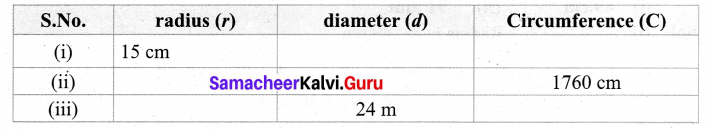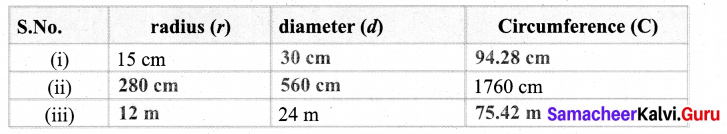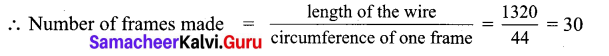# Samacheer Kalvi 7th Maths Solutions Term 2 Chapter 2 Measurements Ex 2.1

Students can Download Maths Chapter 2 Measurements Ex 2.1 Questions and Answers, Notes Pdf, Samacheer Kalvi 7th Maths Book Solutions Guide Pdf helps you to revise the complete Tamilnadu State Board New Syllabus and score more marks in your examinations.

## Tamilnadu Samacheer Kalvi 7th Maths Solutions Term 2 Chapter 2 Measurements Ex 2.1

Samacheer Kalvi 7th Maths Term 2 Question 1.
Find the missing values in the following table for the circles with radius (r), diameter (d) and Circumference (C).Solution:
(i) Given radius r = 15cm
∴ diameter d = 2 × 15 = 30 cm
Circumference C = π d units
= $$\frac { 22 }{ 7 }$$ × 30 = $$\frac { 660 }{ 7 }$$ = 94.28 cm

(ii) Given circumference C = 1760 cm
2πr = 1760
2 × $$\frac { 22 }{ 7 }$$ × r = 1760
r = $$\frac{1760 \times 7}{2 \times 22}$$ = $$\frac{160 \times 7}{2 \times 2}$$ = 40 × 7 = 280 cm
diameter = 2 × r
= 2 × 280 = 560 cm

(iii) diameter d = 24m
radius r = $$\frac { d }{ 2 }$$ = $$\frac { 24 }{ 2 }$$ = 12 m
Circumference C = 2 π r units
= 2 × $$\frac { 22 }{ 7 }$$ × 12 = $$\frac { 528 }{ 7 }$$ = 75.4 m

Tabulating the results7th Maths 2.1 Question 2.
Diameters of different circles are given below. Find their circumference (Take π = $$\frac { 22 }{ 7 }$$ )
(i) d = 70cm
(ii) d = 56m
(iii) d = 28mm
Solution:
(i) Diameter d = 70 cm
Circumference C = π d units = $$\frac { 22 }{ 7 }$$ × 70 = 22 × 10 = 220 cm

(ii) Diameter d = 56 m
Circumference = π d units
= $$\frac { 22 }{ 7 }$$ × 56 = 22 × 8 = 176 m

(iii) Diameter d = 28 mm
Circumference C = π d units = $$\frac { 22 }{ 7 }$$ × 28 = 22 × 4 = 88 mm

7th Maths Guide Term 2 Question 3.
Find the circumference of the circles whose radii are given below.
(i) 49 cm
(ii) 91 mm
Solution:
Circumference C = 2 π r units = 2 × $$\frac { 22 }{ 7 }$$ × 49 = 2 × 22 × 7
= 44 × 7 = 308 cm

(ii) Radius r = 91 mm
Circumference C = 2 π r units
= 2 × $$\frac { 22 }{ 7 }$$ × 91 = 2 × 22 × 13 = 44 × 13 = 572 mm

Samacheer Kalvi 7th Maths Book Answers Question 4.
The diameter of a circular well is 4.2 m. What is its circumference?
Solution:
Given the diameter d = 4.2 m
Circumference C = π d units = $$\frac { 22 }{ 7 }$$ × 4.2 m = 22 × 0.6 = 13.2 m

Samacheer Kalvi 7th Maths Question 5.
The diameter of the bullock cart wheel is 1.4 m. Find the distance covered by it in 150 rotations?
Solution:
Diameter of the bullock cart wheel d= 1.4 m
Distance covered in 1 rotation = Its circumference
= π d units = $$\frac { 22 }{ 7 }$$ × 1 .4 m = 22 × 0.2 = 4.4 m
Distance covered in one rotation = 4.4 m
Distance covered in 150 rotations = 4.4 × 150 = 660.0
Distance covered in 150 rotations = 660 m

Samacheer Kalvi 7th Maths Book Term 2 Question 6.
A ground is in the form of a circle whose diameter is 350 m. An athlete makes 4 revolutions. Find the distance covered by the athlete.
Solution:
Diameter of the ground d = 350 m
Distance covered in 1 revolution = Circumference of the circle
= π d units = $$\frac { 22 }{ 7 }$$ × 350 m = 22 × 50 = 1100 m
Distance covered in 1 rotation = 1100 m
Distance covered in 4 revolutions = 1100 × 4 = 4400 m

Samacheer Kalvi 7th Maths Solutions Question 7.
A wire of length 1320 cm is made into circular frames of radius 7 cm each. How many frames can be made?
Solution:
Length of the wire = 1320 cm
Radius of each circular frame = 7cm
Circumference of the frame 2 π r units = 2 × $$\frac { 22 }{ 7 }$$ × 7 cm = 2 × 22 = 44 cm7th Maths Samacheer Kalvi Question 8.
A Rose garden is in the form of circle of radius 63 m. The gardener wants to fence it at the rate of ₹ 150 per metre. Find the cost of fencing?
Solution:
Radius of the garden r = 63 m
Circumference of the garden = 2 π r units = 2 × $$\frac { 22 }{ 7 }$$ × 63 m = 2 × 22 × 9 = 396 m
Cost of fencing 1 meter = ₹ 150
Cost of fencing 396 meter = ₹ 150 × 396 = ₹ 59,400
∴ Cost of fencing the garden = ₹ 59,400

Objective Type Questions

7th Maths Term 2 Question 9.
Formula used to find the circumference of a circle is
(i) 2πr units
(ii) πr2 + 2r units
(iii) πr2 sq. units
(iv) πr3 cu. units
(i) 2πr units

Samacheer Kalvi 7th Maths Solution Question 10.
In the formula, C = 2πr, ‘r’ refers to
(i) circumference
(ii) area
(iii) rotation

Samacheer Kalvi 7th Maths Books Answers Question 11.
If the circumference of a circle is 82π, then the value of ‘r’ is
(i) 41cm
(ii) 82 cm
(iii) 21cm
(iv) 20 cm